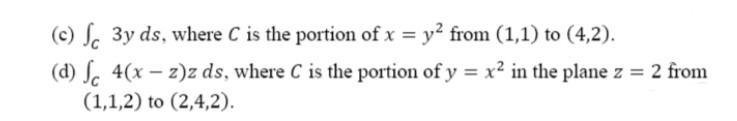### Create an Account

Already have account?

### Forgot Your Password ?

Home / Questions / (0) Sc 3y ds, where C is the portion of x = y2 from (1,1) to (4,2). (d) Sc 4(x – z)z ds, w...

# (0) Sc 3y ds, where C is the portion of x = y2 from (1,1) to (4,2). (d) Sc 4(x – z)z ds, where C is the portion of y = x2 in the plane z = 2 from (1,1,2) to (2,4,2).

(0) Sc 3y ds, where C is the portion of x = y2 from (1,1) to (4,2). (d) Sc 4(x – z)z ds, where C is the portion of y = x2 in the plane z = 2 from (1,1,2) to (2,4,2).Apr 15 2021 View more View Less

#### Answer (Solved)Subscribe To Get Solution